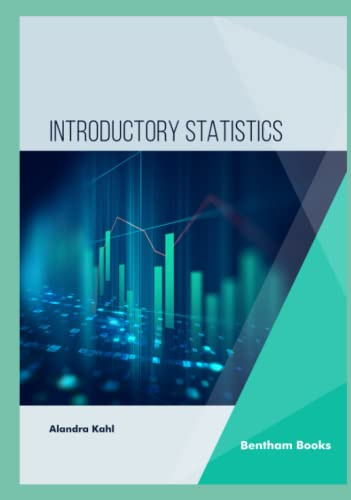# Introductory Statistics

• Length: 178 pages
• Edition: 1
• Publisher:
• Publication Date: 2023-04-14
• ISBN-10: 9815123149
• ISBN-13: 9789815123142
Description

This textbook is a primer for students on statistics. It covers basic statistical operations, an introduction to probability, distributions and regression. The book is divided into a series of 10 chapters covering a basic introduction to common topics for beginners.

The goal of the book is to provide sufficient understanding of how to organize and summarize datasets through descriptive and inferential statistics for good decision-making. A chapter on ethics also informs readers about best practices for using statistics in research and analysis.

Topics covered:

1. Introduction to Statistics
2. Summarizing and Graphing
3. Basic Concepts of Probability
4. Discrete Random Variables
5. Continuous Random Variables
6. Sampling Distributions
7. Estimation
8. Hypothesis Testing
9. Correlation and Regression
10. Ethics